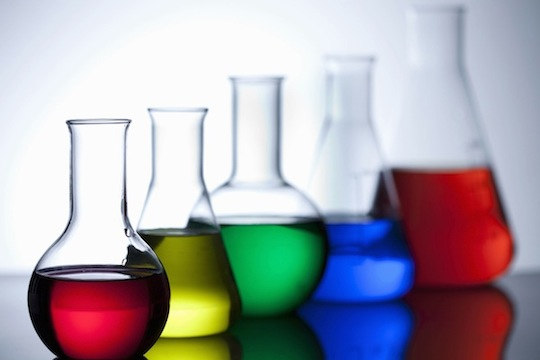# Project Of Christopher Boo

Chemistry Level 2There are two rubidium isotopes occurring in nature: $\text{Rb}^{85}$, which has a molar mass of $84.91$, and $\text{Rb}^{87}$, whose molar mass is $86.92$. The atomic mass of rubidium is $85.47$. What is the percentage of $\text{Rb}^{87}$ out of the total existing $\text{Rb}?$

×